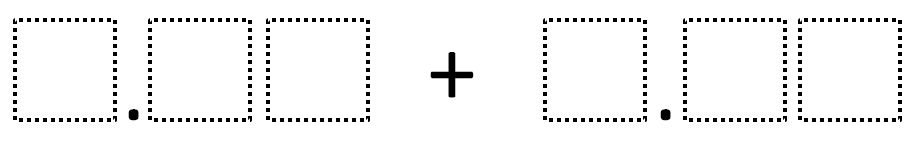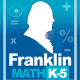Home > Grade 5 > Adding Decimals (Elementary)

# Adding Decimals (Elementary)

Directions: Using the digits 1 to 9 at most one time each, fill in the boxes to make the smallest (or largest) sum.### Hint

What number does each box represent?

### Answer

Thanks to Coen and Robert Lewis for their two answers of 1.35 + 2.46 and 1.46 + 2.35. This also implies that 2.36 + 1.45 and other rearrangements are possible.

Source: Robert Kaplinsky

## Multiplying Decimals to Make a Whole Number Product

Directions: Using the digits 1 to 9 at most one time each, fill in the …

### 6 comments

1.answer is: 1.35 + 2.46

2.Or … 1.46 + 2.35 😎

•Thanks! I added your answers to the problem.

3.My students tried this and, according to the instructions, we could use numbers from 1 to 9. Your solutions use the digits 1 through 6 only. For the largest sum my students got 9.75 + 8.64 and other combinations keeping the digits in the same place values to get the sum of 18.39. Thanks 🙂

4.Just thinking an off shoot of this problem that might bring some different place value discussion to the conversation with children would be to move move the decimal point in the second addend.
For example, use the same exact directions but use the frame..
_._ _ + ._ _ _
or
_._ _ + _ _ ._

5.Pamela Quintern

smallest= 1.35+2.46 to make the sum 3.81 while the largest sum can be created by adding 9.75+8.64 to give 18.39